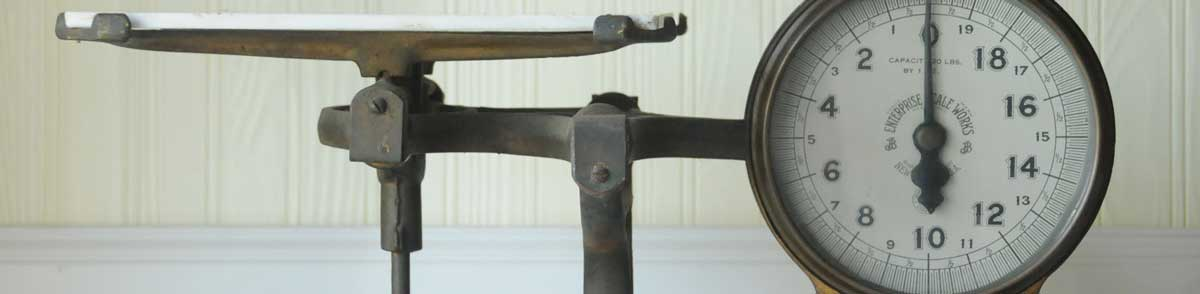# Pounds to Grams Conversion

Enter the weight in pounds below to get the value converted to grams.

lb
oz
Results in Grams:1 lb = 453.59237 g
Do you want to convert grams to pounds?

## How to Convert Pounds to GramsTo convert a pound measurement to a gram measurement, multiply the weight by the conversion ratio.

Since one pound is equal to 453.59237 grams, you can use this simple formula to convert:

grams = pounds × 453.59237

The weight in grams is equal to the pounds multiplied by 453.59237.

For example, here's how to convert 5 pounds to grams using the formula above.
5 lb = (5 × 453.59237) = 2,267.96185 g

## Pounds

One pound is defined as a unit of mass/weight equal to 16 ounces, or 0.45359237 kilograms. One pound is equal to 7,000 grains in the avoirdupois or apothecaries' systems.

The pound is a US customary and imperial unit of weight. A pound is sometimes also referred to as a common ounce. Pounds can be abbreviated as lb, and are also sometimes abbreviated as lbs, lbm, or #. For example, 1 pound can be written as 1 lb, 1 lbs, 1 lbm, or 1 #.

## Grams

A gram is the mass/weight equal to 1/1,000 of a kilogram and is roughly equivalent to the mass of one cubic centimeter of water.

The gram, or gramme, is an SI unit of weight in the metric system. Grams can be abbreviated as g; for example, 1 gram can be written as 1 g.

## Pound to Gram Conversion Table

Pound measurements converted to grams
Pounds Grams
1 lb 453.59 g
2 lb 907.18 g
3 lb 1,361 g
4 lb 1,814 g
5 lb 2,268 g
6 lb 2,722 g
7 lb 3,175 g
8 lb 3,629 g
9 lb 4,082 g
10 lb 4,536 g
11 lb 4,990 g
12 lb 5,443 g
13 lb 5,897 g
14 lb 6,350 g
15 lb 6,804 g
16 lb 7,257 g
17 lb 7,711 g
18 lb 8,165 g
19 lb 8,618 g
20 lb 9,072 g
21 lb 9,525 g
22 lb 9,979 g
23 lb 10,433 g
24 lb 10,886 g
25 lb 11,340 g
26 lb 11,793 g
27 lb 12,247 g
28 lb 12,701 g
29 lb 13,154 g
30 lb 13,608 g
31 lb 14,061 g
32 lb 14,515 g
33 lb 14,969 g
34 lb 15,422 g
35 lb 15,876 g
36 lb 16,329 g
37 lb 16,783 g
38 lb 17,237 g
39 lb 17,690 g
40 lb 18,144 g

## References

1. Encyclopædia Britannica, Avoirdupois weight, https://www.britannica.com/science/avoirdupois-weight